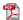Useful formulas for RF related conversionsTypical EMC Related Formulas

Term Conversions
 Convert: dBuV dBmV dBV dBuA dBmA dBA dBm dBW uV mV V uA mA A mW W To: dBuV dBmV dBV dBuA dBmA dBA dBm dBW uV mV V uA mA A mW W Impedance: ohms

dBmW = dBµV - 107
The constant in the above equation is derived as follows.  Power is related to voltage according to Ohm's law.  The Log10 function is used for relative (dB) scales, so applying the logarithmic function to Ohm's law, simplifying, and scaling by ten (for significant figures) yields:
P = V2/ R

10Log10[P] = 20Log10[V] - 10Log10

Note, the resistance of 50 used above reflects that RF systems are matched to 50Ω. Since RF systems use decibels referenced from 1 mW, the corresponding voltage increase for every 1 mW power increase can be calculated with another form of Ohm's law:
V = (PR)0.5 = 0.223 V = 223000 µV

Given a resistance of 50Ω and a power of 1 mW
20Log10[223000 µV] = 107 dB

The logarithmic form of Ohm's law shown above is provided to describe why the log of the corresponding voltage is multiplied by 20.

dBmW/m2 = dBµV/m - 115.8
The constant in this equation is derived following similar logic. First, consider the poynting vector which relates the power density (W/m2)to the electric field strength (V/m) by the following equation:
P=|E|2

Where η is the free space characteristic impedance equal to 120p Transforming this equation to decibels and using the appropriate conversion factor to convert dBW/m2 to dBmW/m2 for power density and dBV/m to dBµV/m for the electric field, the constant becomes 115.8
dBµV/m = dBµV + AF

Where AF is the antenna factor of the antenna being used, provided by the antenna manufacturer or a calibration that was performed within the last year.
V/m = 10{[(dBµV/m)-120]/20}

Not much to this one, just plug away!
dBµA/m = dBµV/m - 51.5

To derive the constant for the above equation, simply convert the characteristic impedance of free space to decibels, as shown below:
20Log10[120p] = 51.5

A/m = 10{[(dBµA/m)-120]/20}

As above, simply plug away.
dBW/m2 = 10Log10[V/m - A/m]

A simple relation to calculate decibel-Watts per square meter.
dBmW/m2= dBW/m2 + 30

The derivation for the constant in the above equation comes from the decibel equivalent of the factor of 1000 used to convert W to mW and vice versa, as shown below:
10Log10 = 30

dBpT = dBµA/m + 2.0

In this equation, the constant 2.0 is derived as follows. The magnetic flux density, B in Teslas (T), is related to the magnetic field strength, H in A/m, by the permeability of the medium in Henrys per meter (H/m). For free space, the permeability is given as...
µo = 4p x 10-7 H/m

Converting from T to pT and from A/m to µA/m, and deriving the Log, the constant becomes:
240 - 120 + 20Log10[4p x 10-7] = 2.0

dBpT = dBuV + dBpT/uV + Cable Loss
dBuV/m = dBpT + 49.5 dB

View our other RF Conversion formula sheets:
Antenna Factor and Gain Calculations
dBm to Volts to Watts conversion
Magnetic field conversions
Field Intensity Calculation
Antenna Beamwidth Coverage Calculations
Frequency and Wavelength Calculator
VSWR Calculations

Contact Details

A.H. Systems, inc.
9710 Cozycroft Ave.
Chatsworth, CA 91311

P: (818) 998-0223

F: (818) 998-6892

E: Email

Product Showcase

Antenna Kit - AK-40G• 20 Hz to 40 GHz
• FCC, MIL-STD and CISPR Testing
• Three Year Warranty

Constantly changing for today's dynamic environment, A.H. Systems presents the proven line of Antenna Kits. Designed to meet the needs of your various testing requirements.